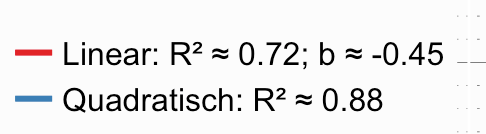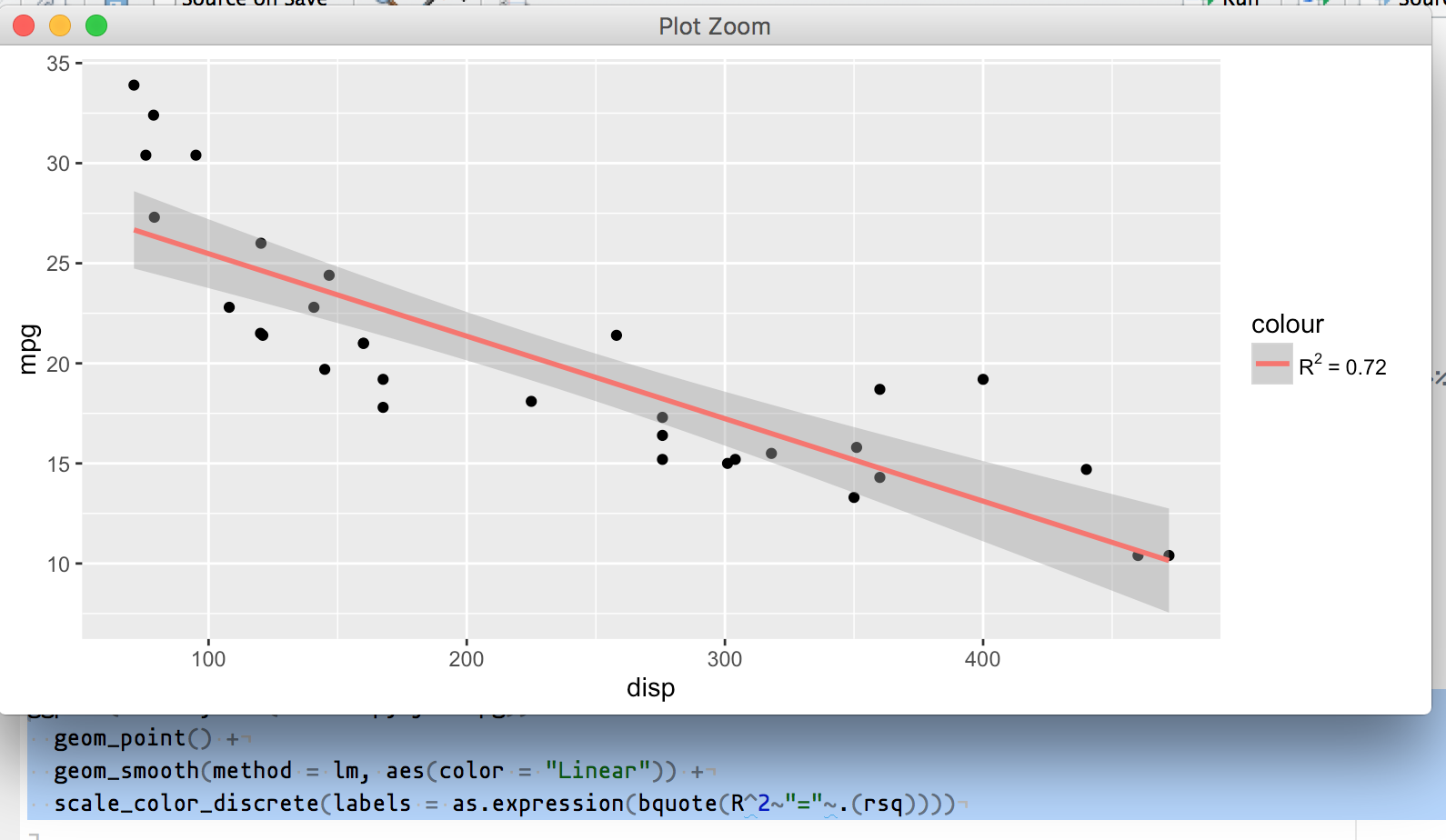# R / ggplot2: Evaluate object inside expression

Example code:

``````rsq <- round(cor(mtcars\$disp, mtcars\$mpg)^2, 2) # rsq = 0.72

ggplot(mtcars, aes(x = disp, y = mpg)) +
geom_point() +
geom_smooth(method = lm, aes(color = "Linear")) +
scale_color_discrete(labels = expression(paste("R"^2, " = ", rsq)))
``````

I would like the legend to be displayed as `R² = 0.72`.
I know I can just use the unicode symbol for ² to get the superscript, but in general I think there has to be a way to combine math expressions and calculated values stored in objects.

I have tried to play around with `eval` and various combinations of `paste`, but it seems I keep running into the same problem.

Edit #1:
I tried using `bquote` according to this answer like this:

`scale_color_discrete(labels = bquote(R^2 == .(rsq)))`

Turns out that only renders the legend as `==`.

Edit #2:
Even though the answer below works, it seems… very inconvenient for more complex expressions, like this:I'm still hoping for a simpler solution.

Turns out the `bquote` thing was close.
This works (although it feels… suboptimal):

``````  scale_color_discrete(labels = as.expression(bquote(R^2~"="~.(rsq))))
``````

Also working:

`````` scale_color_discrete(labels = as.expression(bquote(R^2 == .(rsq))))
``````

Apparently the `~` are required to "paste" the elements together, without actually `paste()`ing them? And `as.expression` does what `expression` couldn't. I'm not sure what exactly is going on, but alas, it works:Thanks a lot, Peter Dalgaard!

I noticed that, as of version 3.3.2 of the ggplot2 package, both `scale_color_discrete()` and `scale_color_manual()` now accept `bquote()` labels directly. Presumably this change applies more broadly to other `scale_` functions but I haven't tested more widely.

Simpler approaches like `geom_line(aes(color = bquote(...)))` and `geom_line(aes(color = as.expression(bquote(...))))` are still rejected as having invalid aesthetics, though.

``````data = data.frame(x = seq(0, 5, length.out = 50))
data\$exp1 = 1 - exp(-data\$x)
data\$exp0.5 = 1 - exp(-0.5 * data\$x)
ggplot(data, aes(x = x)) + geom_line(aes(y = exp1, color = "exp1")) +
geom_line(aes(y = exp0.5, color = "exp0.5")) +
scale_color_manual(labels = c(bquote(1 - e^-x), bquote(1 - e^"-0.5x")), values = c("blue", "green")) + # or scale_color_discrete(labels = c(bquote(1 - e^-x), bquote(1 - e^"-0.5x")))
labs(y = "y") + theme(legend.position = c(0.8, 0.15))
``````